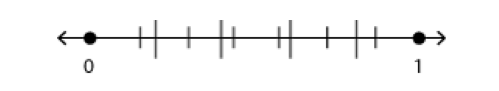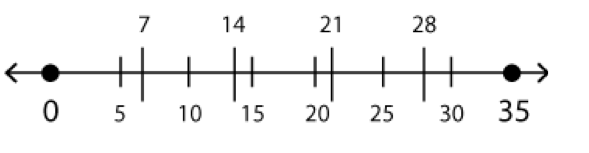# Reorient Your View on Math Problems - Part 2

by , Oct 26, 2013In the first part of this article, we talked about how GMAT quant problems are often written to imply a certain approach or solution path that is not actually the best way to do the problem. We want to reorient our view in order to pick an easier, more efficient setup or solution (if at all possible).

We finished off with a homework assignment; heres the problem I gave you (from the free problems that come with the GMATPrep software):

* If [pmath]{x/y}={2/3}[/pmath], then [pmath]{x-y}/x[/pmath] =

(A)[pmath]-1/2[/pmath]

(B)[pmath]-1/3[/pmath]

(C)[pmath]1/3[/pmath]

(D)[pmath]1/2[/pmath]

(E) [pmath]5/2[/pmath]

The answers are fractions but they arent horrible fractions. They give me a value for [pmath]x/y[/pmath]. The question is kind of annoying though, because the form doesnt match [pmath]x/y[/pmath].

Or does it? Is there any way for me to rearrange that thing to make it look more like [pmath]x/y[/pmath]?

Yes! Check it out:

[pmath]{x-y}/x=x/x-y/x=1-y/x[/pmath]

Now, how did I know to do that? Ive actually seen another problem with the same shortcut: split the numerator into two fractions. The first time I saw that other problem, though, the way I figured it out was that whole Well, this is annoying, why did they give it to me that way! And so I started looking at it differently and asking myself some questions:

They gave me a value for [pmath]x/y[/pmath]. But the question doesnt give me [pmath]x/y[/pmath]. Is there any way I can make [pmath]x/y[/pmath]? There is an x on top and a y on the bottom; what if I put those two together?

Oh, yeah, I see! Its totally legal to split the numerator and get two separate fractions, so that would give me [pmath]x/y[/pmath]for one of the fractions. Does that make my life any easier, though?

The other fraction just turns into 1! Thats fantastic! I know what Im doing now.

Et voil! I know that [pmath]x/y=2/3[/pmath], so [pmath]{y/x}={3/2}[/pmath]. Plug that in and get 1 1.5 = -0.5.

Note that its easier to add and subtract in decimal (or percent) form, so if fractions can be converted easily (as [pmath]3/2[/pmath] can), then consider doing the subtraction in decimal form. You already know that it will be easy to convert back into the final answer because look at the answer optionstheyre all easy fractions to convert.

Quick! Glance at the answer choices for the above problem. If you did no work at all and had 1 second to make a guess, which answer would you NOT pick?

You wouldnt want to pick the last answer. (Im deliberately not typing the letter so that your eye cant pick it up when trying to answer my question two sentences ago!) Four of the answers come in obvious pairs; that is, theyre the same except for the sign (positive or negative). The chances are very good, then, that you need to be very careful about signs when doing the work and that the odd answer out will not be the correct answer. Why?

Because one of the traps this problem is setting is the sign; some number of people will drop the negative by accident, and wind up with (D) as the answer instead of (A). That trap doesnt exist for answer (E) because it doesnt have an opposite sign counterpart. So, if you have to guess, dont pick it.

Ready to try another? Here you go (once again, this is from the free problem set that comes with GMATPrep):* On the number line above, the segment from 0 to 1 has been divided into fifths, as indicated by the large tick marks, and also into sevenths, as indicated by the small tick marks. What is the least possible distance between any two of the tick marks?

(A)[pmath]1/70[/pmath]

(B)[pmath]1/35[/pmath]

(C)[pmath]2/35[/pmath]

(D)[pmath]1/12[/pmath]

(E) [pmath]1/7[/pmath]

Fifths and sevenths? No, this problem isnt annoying at all.(Yes, thats my sarcasm voice.)

Remember our four process steps from the first half of this article? What was the first one again?

Glance! In this case, glance at those answer choices. Theyre fractions. Why is that useful? Often, when something is presented in terms of fractions or percentages, you can either pick your own number (if no real numbers are given) or you can adjust the numbers given to easier numbers (as long as all relationships are kept intact).

In this case, we can't pick any random numbers that we like because they do give some parameters. Those parameters, though, are incredibly annoying: [pmath]1/5, 2/5, 3/5[/pmath], and [pmath]4/5[/pmath] are not too bad, but then we have [pmath]1/7, 2/7, 3/7[/pmath], and so on. Yuck!

Why couldnt they have given me whole numbers instead of fractions?

Hey, wait a second why cant I turn these into whole numbers instead of fractions myself? The answers are also in fraction form, so I can!!

If you were going to do the real math, youd have to find common denominators for 5 and 7. Instead, just make the whole number line 0 to 35!Now, there are tick marks at the fifths (7, 14, 21, 28) and at the 7ths (5, 10, 15, 20, 25, 30). Find the two, one from each set, that are closest together.

Maybe you pick 14 and 15, for a difference of 1. Or maybe you pick 20 and 21 for the same difference of 1.

The answer isnt just 1 thoughits 1 out of 35, or [pmath]1/35[/pmath].

One more intricacy: the problem asks for the least possible distance. How can you be sure that [pmath]1/70[/pmath] (the only smaller answer) is not a possible distance?

If youre checking all the numbers in fraction form, then you really cant be 100% sure till you check them all. Yuck. If youre doing the problem in integer form, though, then you know you cant get a smaller distance than 1, because theyre all integers. At the least, they have to be 1 apart. In addition, the denominator has to be 35, because thats the new length of the line. So you know it cant get any smaller than [pmath]1/35[/pmath].

This last problem nicely illustrates the concept that weve been discussing: whoever wrote the question is implying a certain path and part of the test is whether you just start walking down it or whether you glance around first to choose the best path for you. In this case, they set us up with fractions, but we arent required to follow that path. We can turn this thing into integers instead. And who wouldnt rather work with integers?

## Key Takeaways for Reorienting Your View

(1) If a problem seems to imply a certain path, be skeptical. After all, the test writers arent in the business of helping you get a better score on the test! Take a step back and choose an approach based on your own knowledge and strengths.

(2) Dont just dive in! Think about what youve got and where youre trying to go before you pick a path to get there. Remember:

1. Glance